GMAT  >  Solved Examples - Beyond Equations: Inequalities & Absolute Value

# Solved Examples - Beyond Equations: Inequalities & Absolute Value - Notes | Study Quantitative for GMAT - GMAT

 1 Crore+ students have signed up on EduRev. Have you?

Section - 1
Draw the following inequalities on the number line provided:

Ques 1: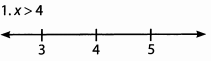Ans: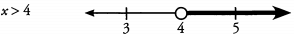Ques 2: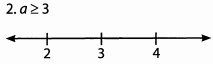Ans: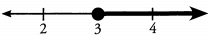Ques 3: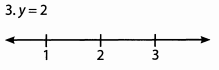Ans: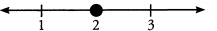Ques 4: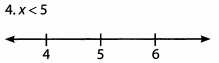Ans: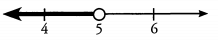Ques 5: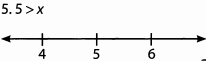Ans: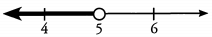Section - 2
Ques 6: a is less than b.
Ans:
a is less than b.
a < b

Ques 7: Five times x is greater than 10.
Ans:
Five times x is greater than 10.
5x > 10

Ques 8: Six is less than or equal to 4x.
Ans:
Six is less than or equal to 4x.
6 < 4x

Ques 9: The price of an apple is greater than the price of an orange.
Ans:
The price of an apple is greater than the price of an orange.
Let a = the price of an apple
Let o = the price of an orange
a > o
Note: In this problem, we set up our variables to refer to prices— not the number of apples and oranges.

Ques 10: The total number of members is at least 19.
Ans:
The total number of members is at least 19.
Let m — the number of members
m > 19

Section - 3
Solve the following inequalities.
Ques 11: x + 3 ≤- 2
Ans: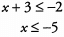Ques 12: f - 4 ≤ 13
Ans: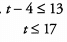Ques 13: 3b≥ 12
Ans: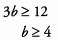Ques 14: -5x > 25
Ans: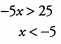Ques 15: -8<-4y
Ans: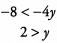Section - 4
Solve the following equations.
Ques 16: 2z + 4 ≥-18
Ans: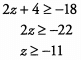Ques 17: 7y - 3 ≤ 4y + 9
Ans: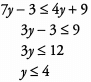Ques 18: b/5 ≤ 4
Ans: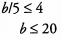Ques 19: d + 3/2<8
Ans: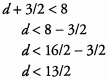Ques 20: 4x/ 7 ≤ 15 + x
Ans: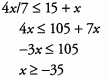Section - 5
Solve the following inequalities.
Ques 21: 3 ( x - 7 ) ≥ 9
Ans: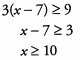Ques 22: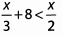Ans: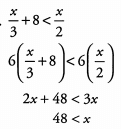Ques 23: 2x - 1.5 > 7
Ans: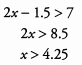Ques 24: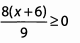Ans: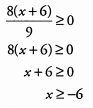Ques 25: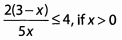Ans: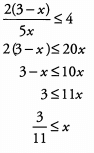The document Solved Examples - Beyond Equations: Inequalities & Absolute Value - Notes | Study Quantitative for GMAT - GMAT is a part of the GMAT Course Quantitative for GMAT.
All you need of GMAT at this link: GMAT

## Quantitative for GMAT

88 videos|99 docs|165 tests

## Quantitative for GMAT

88 videos|99 docs|165 tests

Track your progress, build streaks, highlight & save important lessons and more!

,

,

,

,

,

,

,

,

,

,

,

,

,

,

,

,

,

,

,

,

,

;